## Example Questions

### Example Question #1 : Acute / Obtuse Triangles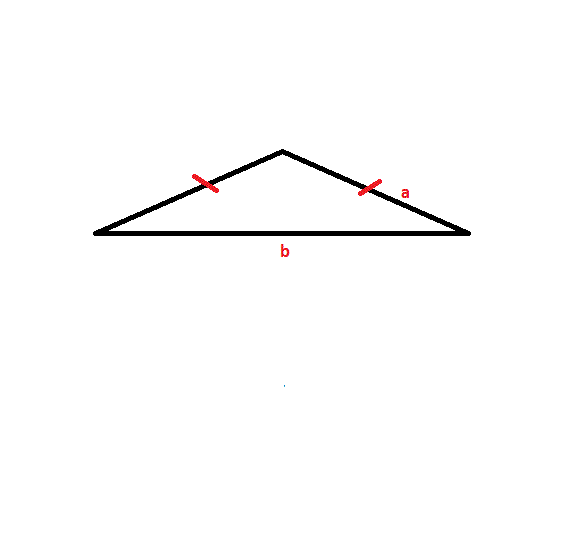The obtuse Isosceles triangle shown above has two sides with lengthand one side length. The length of side. The length of side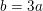. Find the perimeter of the triangle.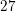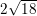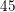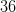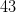Explanation:

To solve this problem, apply the formula: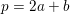Side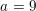, sidemust equal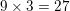Since this is an Isosceles triangle, there must be two sides with a length of.

Thus, plug in each side lengths value to find the solution: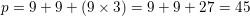Tired of practice problems?

Try live online GRE prep today.